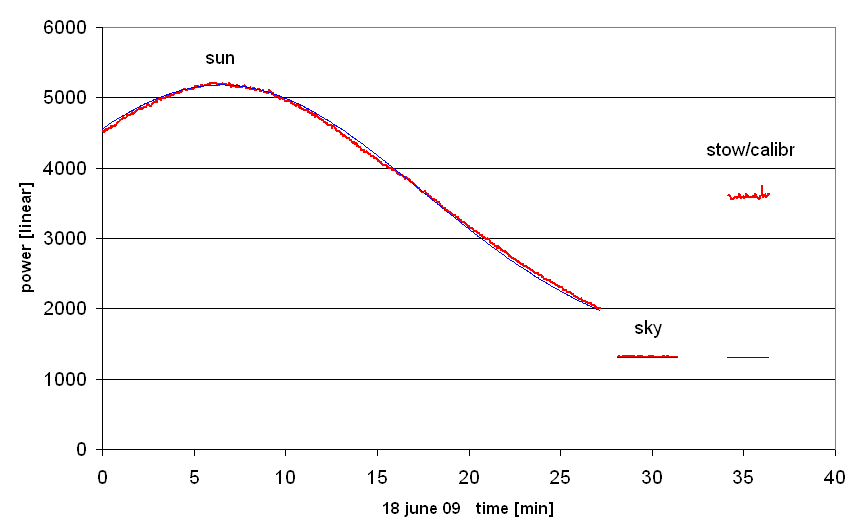The Antenna Pattern

Joachim Köppen Strasbourg 2010

Our first solar observation on 18 june 2009 was a Drift scan in which we let the Sun pass through a position where the fixed telescope is pointing. The data recorded shows therefore a peak flux when the Sun moved through the main lobe of the antenna:We waited until the signal was much lower than the maximum value, then we moved the telescope to a position at the same elevation, but at about 90° to the East of the Sun to measure the background by observing an empty patch of the sky. Finally, we moved to stow position for flux calibration with the library's wall.

By fitting a gaussian curve (the thin blue curve) to the solar data, we determine its width sigma = 11.04 minutes of time. As the Sun was at declination +23.40°, it moved across the sky with angular speed of 0.25°/min*cos(declination), so we can express sigma in degrees. The half-power beam width of the gaussian curve is given by HPBW = 2.3548*sigma. Taken all together, this gives a HPBW of our antenna of 5.95°.

But we also notice that the measured curve deviates from the gaussian curve slightly, but significantly ...!

A more recent observation - in the same manner - gave HPBW = 6.2°

last update: Apr. 2010 J.Köppen